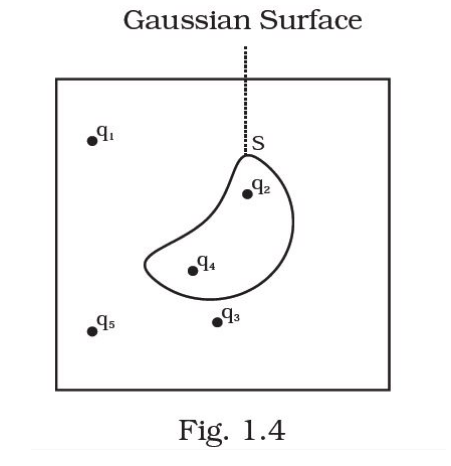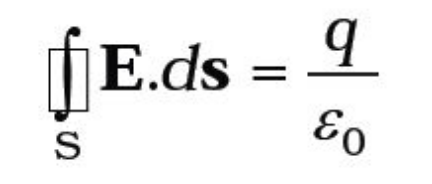Deepak Scored 45->99%ile with Bounce Back Crack Course. You can do it too!

# Five charges q1, q2, q3, q4, and q5

Question:

Five charges q1q2q3q4, and q5 are fixed at their positions as shown in Fig. 1.4. S is a Gaussian surface.The Gauss’s law is given byWhich of the following statements is correct?

(a) on the LHS of the above equation will have a contribution from q1qand q3 while on the RHS will have a contribution from q2 and q4 only.

(b) E on the LHS of the above equation will have a contribution from all charges while q on the RHS will have a contribution from q2 and q4 only.

(c) E on the LHS of the above equation will have a contribution from all charges while q on the RHS will have a contribution from q1q3 and q5 only.

(d) Both E on the LHS and on the RHS will have contributions from q2 and q4 only.

Solution:

According to Gauss' theorem, the term q on the right side of the equation includes the sum of all charges enclosed by the surface (irrespective of the position of the charges inside the surface).

If the surface is so chosen that there are some charges inside and some outside, the electric field on the left side of equation will be due to all charges (both inside and outside)

Here, E on LHS of the above equation will have a contribution from all charges while on the RHS will have a contribution from charges q2 and q4 only.